2022: SklogWiki celebrates 15 years on-line

# Monte Carlo in the microcanonical ensemble

## Integration of the kinetic degrees of freedom

Consider a system of$\left. N \right.$ identical particles, with total energy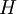$\left. H \right.$ given by: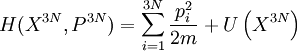$H(X^{3N},P^{3N}) = \sum_{i=1}^{3N} \frac{p_i^2}{2m} + U \left( X^{3N} \right)$; (Eq.1)

where:

•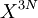$\left. X^{3N} \right.$ represents the 3N Cartesian position coordinates of the particles
•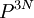$\left. P^{3N} \right.$ stands for the the 3N momenta.

The first term on the right hand side of (Eq. 1) is the kinetic energy, whereas the second term is the potential energy (a function of the positional coordinates).

Now, let us consider the system in a microcanonical ensemble; let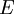$\left. E \right.$ be the total energy of the system (constrained in this ensemble).

The probability,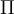$\left. \Pi \right.$ of a given position configuration$\left. X^{3N} \right.$, with potential energy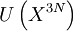$U \left( X^{3N} \right)$ can be written as: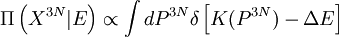$\Pi \left( X^{3N}|E \right) \propto \int d P^{3N} \delta \left[ K(P^{3N}) - \Delta E \right]$ ; (Eq. 2)

where:

•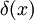$\left. \delta(x) \right.$ is the Dirac's delta function
•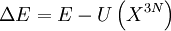$\Delta E = E - U\left(X^{3N}\right)$.

The Integral in the right hand side of (Eq. 2) corresponds to the surface of a 3N-dimensional (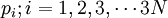$p_i; i=1,2,3,\cdots 3N$) hyper-sphere of radius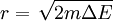$r = \left. \sqrt{ 2 m \Delta E } \right.$ ; therefore: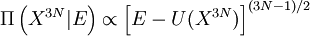$\Pi \left( X^{3N}|E \right) \propto \left[ E- U(X^{3N}) \right]^{(3N-1)/2}$.

See Ref. 1 for an application of Monte Carlo simulation using this ensemble.